## Concave Mirrors

Convex Mirrors

We give the definition of convex mirrors in previous sections. Now we will examine the reflection of light from this type of mirrors and image formation in convex mirrors. Let’s start with the reflection of light with special examples.

1. In convex mirrors, ray coming parallel to the principal axis goes after reflection as if it comes from the focal point of the mirror.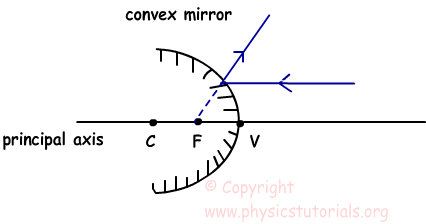2. If the extension of the coming ray passes from the focal point, it travels parallel to the principal axis after reflection from the mirror.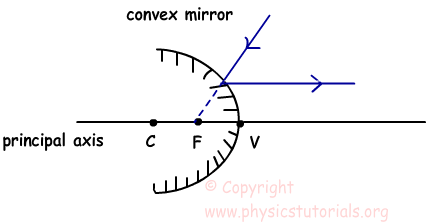3. If the extension of coming ray passes from the center of the curvature, ray reflects from the mirror and travels on itself. As we said before line joining the mirror to the center of the curvature is perpendicular to the mirror and normal of the system.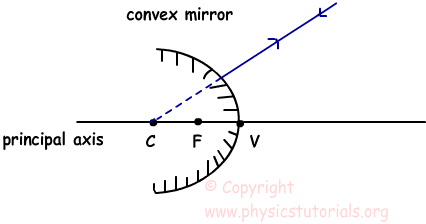4. Ray strikes to the mirror at vertex, reflects from the mirror with the same angle it comes because, principal axis is the normal of the system.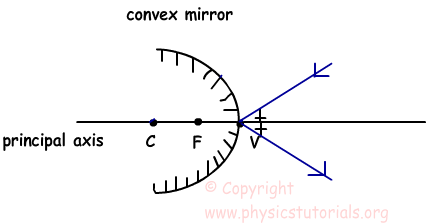Example: Look at the path of the ray R in the given picture, f1 is the focal length of the convex mirror and f2 is the focal length of the concave mirror. Find the distance between then mirrors in terms of f1 and f2.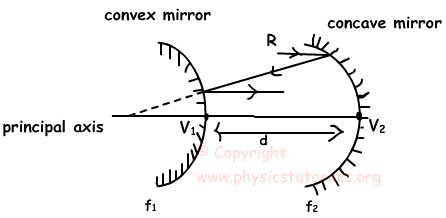Ray coming parallel to the principal axis must go to the focal point of the concave mirror, after reflecting from the concave mirror ray strikes to the convex mirror and goes parallel to the principal axis. This shows us that; ray coming from concave mirror goes to the focal point of the convex mirror. Let me show these explanations on the picture.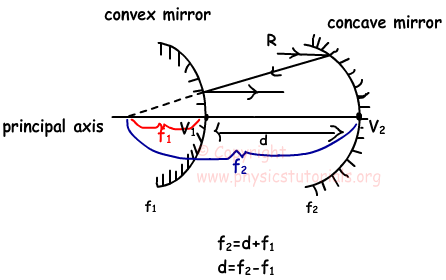Author: